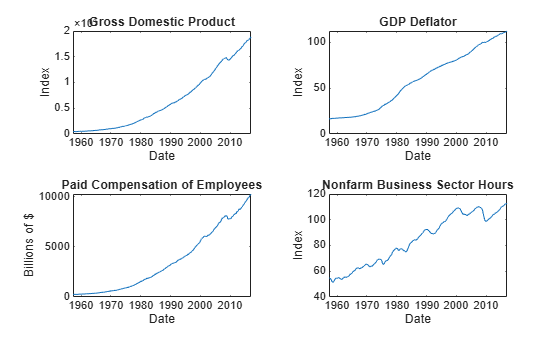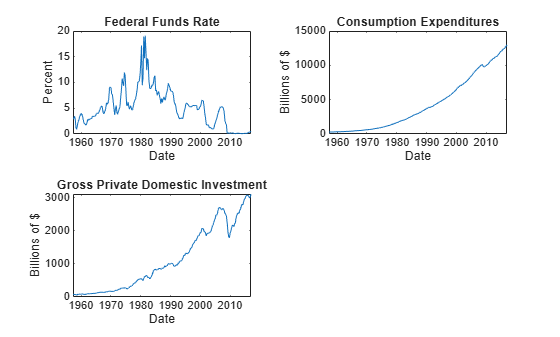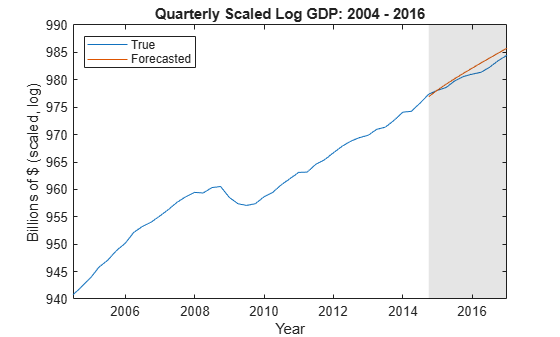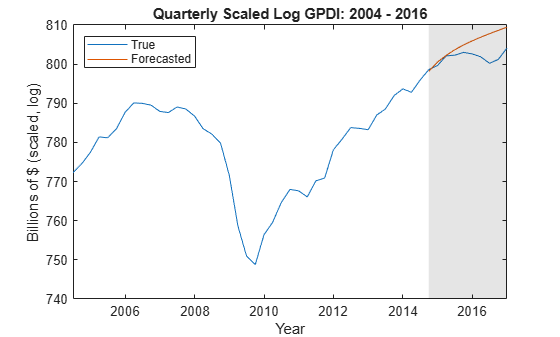# vecm

Create vector error-correction (VEC) model

## Description

The `vecm` function returns a `vecm` object specifying the functional form and storing the parameter values of a (p – 1)-order, cointegrated, multivariate vector error-correction model (VEC((p – 1)) model.

The key components of a `vecm` object include the number of time series (response-variable dimensionality), the number of cointegrating relations among the response variables (cointegrating rank), and the degree of the multivariate autoregressive polynomial composed of first differences of the response series (short-run polynomial), which is p – 1. That is, p – 1 is the maximum lag with a nonzero coefficient matrix, and p is the order of the vector autoregression (VAR) model representation of the VEC model. Other model components include a regression component to associate the same exogenous predictor variables to each response series, and constant and time trend terms.

Another important component of a VEC model is its Johansen form because it dictates how MATLAB® includes deterministic terms in the model. This specification has implications on the estimation procedure and allowable equality constraints. For more details, see Johansen Form and .

Given the response-variable dimensionality, cointegrating rank, and short-run polynomial degree, all coefficient matrices and innovation-distribution parameters are unknown and estimable unless you specify their values by using name-value pair argument syntax. To choose which Johansen form is suitable for your data, then estimate a model containing all or partially unknown parameter values given the data, use `estimate`. To work with an estimated or fully specified `vecm` model object, pass it to an object function. Alternatively, you can create and work with `vecm` model objects interactively by using Econometric Modeler.

## Creation

### Syntax

``Mdl = vecm(numseries,rank,numlags)``
``Mdl = vecm(Name,Value)``

### Description

example

``Mdl = vecm(numseries,rank,numlags)` creates a VEC(`numlags`) model composed of `numseries` time series containing `rank` cointegrating relations. The maximum nonzero lag in the short-run polynomial is `numlags`. All lags and the error-correction term have `numseries`-by-`numseries` coefficient matrices composed of `NaN` values.This shorthand syntax allows for easy model template creation in which you specify the model dimensions explicitly. The model template is suited for unrestricted parameter estimation, that is, estimation without any parameter equality constraints. After you create a model, you can alter property values using dot notation.`

example

``Mdl = vecm(Name,Value)` sets properties or additional options using name-value pair arguments. Enclose each name in quotes. For example, `'Lags',[1 4],'ShortRun',ShortRun` specifies the two short-run coefficient matrices in `ShortRun` at lags `1` and `4`.This longhand syntax allows for creating more flexible models. However, `vecm` must be able to infer the number of series (`NumSeries`) and cointegrating rank (`Rank`) from the specified name-value pair arguments. Name-value pair arguments and property values that correspond to the number of time series and cointegrating rank must be consistent with each other.`

### Input Arguments

expand all

The shorthand syntax provides an easy way for you to create model templates that are suitable for unrestricted parameter estimation. For example, to create a VEC(2) model composed of three response series containing one cointegrating relation and unknown parameter values, enter:

`Mdl = vecm(3,1,2);`
To impose equality constraints on parameter values during estimation, set the appropriate property values using dot notation.

Number of time series m, specified as a positive integer. `numseries` specifies the dimensionality of the multivariate response variable yt and innovation εt.

`numseries` sets the `NumSeries` property.

Data Types: `double`

Number of cointegrating relations, specified as a nonnegative integer. The adjustment and cointegration matrices in the model have `rank` linearly independent columns, and are `numseries`-by-`rank` matrices composed of `NaN` values.

Data Types: `double`

Number of first differences of responses to include in the short-run polynomial of the VEC(p – 1) model, specified as a nonnegative integer. That is, `numlags` = p – 1. Consequently, `numlags` specifies the number of short-run terms associated with the corresponding VAR(p) model.

All lags have `numseries`-by-`numseries` short-run coefficient matrices composed of `NaN` values.

Data Types: `double`

Name-Value Arguments

Specify optional pairs of arguments as `Name1=Value1,...,NameN=ValueN`, where `Name` is the argument name and `Value` is the corresponding value. Name-value arguments must appear after other arguments, but the order of the pairs does not matter.

Before R2021a, use commas to separate each name and value, and enclose `Name` in quotes.

The longhand syntax enables you to create models in which some or all coefficients are known. During estimation, `estimate` imposes equality constraints on any known parameters. Specify enough information for `vecm` to infer the number of response series and the cointegrating rank.

Example: `'Adjustment',nan(3,2),'Lags',[4 8]` specifies a three-dimensional VEC(8) model with two cointegrating relations and nonzero short-run coefficient matrices at lags `4` and `8`.

Short-run polynomial lags, specified as the comma-separated pair consisting of `'Lags'` and a numeric vector containing at most `P` – 1 elements of unique positive integers.

The matrix `ShortRun{j}` is the coefficient of lag `Lags(j)`.

Example: `'Lags',[1 4]`

Data Types: `double`

## Properties

expand all

You can set writable property values when you create the model object by using name-value pair argument syntax, or after you create the model object by using dot notation. For example, to create a VEC(1) model in the H1 Johansen form suitable for simulation, and composed of two response series of cointegrating rank one and no overall time trend term, enter:

```Mdl = vecm('Constant',[0; 0.01],'Adjustment',[-0.1; 0.15],... 'Cointegration',[1; -4],'ShortRun',{[0.3 -0.15 ; 0.1 -0.3]},... 'Covariance',eye(2)); Mdl.Trend = 0;```

Number of time series m, specified as a positive integer. `NumSeries` specifies the dimensionality of the multivariate response variable yt and innovation εt.

Data Types: `double`

Number of cointegrating relations, specified as a nonnegative integer. The adjustment and cointegration matrices in the model have `Rank` linearly independent columns and are `NumSeries`-by-`Rank` matrices.

Data Types: `double`

Corresponding VAR model order, specified as a nonnegative integer. `P` – 1 is the maximum lag in the short-run polynomial that has a nonzero coefficient matrix. Lags in the short-run polynomial that have degree less than `P` – 1 can have coefficient matrices composed entirely of zeros.

`P` specifies the number of presample observations required to initialize the model.

Data Types: `double`

Model description, specified as a string scalar or character vector. `vecm` stores the value as a string scalar. The default value describes the parametric form of the model, for example `"2-Dimensional Rank = 1 VEC(1) Model"`.

Example: `'Description','Model 1'`

Data Types: `string` | `char`

Response series names, specified as a `NumSeries` length string vector. The default is ```['Y1' 'Y2' ... 'YNumSeries']```.

Example: `'SeriesNames',{'CPI' 'Unemployment'}`

Data Types: `string`

Overall model constant (c), specified as a `NumSeries`-by-1 numeric vector.

The value of `Constant`, and whether `estimate` supports equality constraints on it during estimation, depend on the Johansen form of the VEC model.

Example: `'Constant',[1; 2]`

Data Types: `double`

Overall linear time trend (d), specified as a `NumSeries`-by-1 numeric vector.

The value of `Trend`, and whether `estimate` supports equality constraints on it during estimation, depend on the Johansen form of the VEC model.

Example: `'Trend',[0.1; 0.2]`

Data Types: `double`

Cointegration adjustment speeds (A), specified as a `NumSeries`-by-`Rank` numeric matrix.

If you specify a matrix of known values, then all columns must be linearly independent (that is, `Adjustment` must be a matrix of full column rank).

For estimation, you can impose equality constraints on the cointegration adjustment speeds by supplying a matrix composed entirely of numeric values or a mixture of numeric and missing (`NaN`) values.

If `Rank` = `0`, then `Adjustment` is an empty `NumSeries`-by-`0` vector.

For more details on specifying `Adjustment`, see Algorithms.

Example: `'Adjustment',NaN(2,1)`

Data Types: `double`

Cointegration matrix (B), specified as a `NumSeries`-by-`Rank` numeric matrix.

If you specify a matrix of known values, then all columns must be linearly independent (that is, `Cointegration` must be a matrix of full column rank).

`Cointegration` cannot contain a mixture of missing (`NaN`) values and numeric values. Supported equality constraints on the cointegration matrix during estimation depend on the Johansen form of the VEC model.

If `Rank` = `0`, then `Cointegration` is an empty `NumSeries`-by-`0` vector.

For more details on specifying `Cointegration`, see Algorithms.

Example: `'Cointegration',NaN(2,1)`

Data Types: `double`

Impact, or long-run level, matrix (Π), specified as a `NumSeries`-by-`NumSeries` numeric matrix. The rank of `Impact` must be `Rank`.

For estimation of full-rank models (`Rank` = `NumSeries`), you can impose equality constraints on the impact matrix by supplying a matrix containing a mixture of numeric and missing values (`NaN`).

If `1``Rank``NumSeries``1`, then the default value is `Adjustment*Cointegration'`.

If `Rank` = 0, then `Impact` is a matrix of zeros. Consequently, the model does not have an error-correction term.

For more details on specifying `Impact`, see Algorithms.

Example: `'Impact',[0.5 0.25 0; 0.3 0.15 0; 0 0 0.9]`

Data Types: `double`

Constant (intercept) in the cointegrating relations (c0), specified as a `Rank`-by-`1` numeric vector. You can set `CointegrationConstant` only by using dot notation after you create the model.

`CointegrationConstant` cannot contain a mixture of missing (`NaN`) values and numeric values. Supported equality constraints on the cointegration constant vector during estimation depend on the Johansen form of the VEC model.

If `Rank` = `0`, then `CointegrationConstant` is a `0`-by-`1` vector of zeros.

Example: `Mdl.CointegrationConstant = [1; 0]`

Data Types: `double`

Time trend in the cointegrating relations (d0), specified as a `Rank`-by-`1` numeric vector. You can set `CointegrationTrend` only by using dot notation after you create the model.

`CointegrationTrend` cannot contain a mixture of missing (`NaN`) values and numeric values. Supported equality constraints on the cointegration linear trend vector during estimation depend on the Johansen form of the VEC model.

If `Rank` = 0, then `CointegrationTrend` is a `0`-by-`1` vector of zeros.

Example: `Mdl.CointegrationTrend = [0; 0.5]`

Data Types: `double`

Short-run coefficient matrices associated with the lagged response differences, specified as a cell vector of `NumSeries`-by-`NumSeries` numeric matrices.

Specify coefficient signs corresponding to those coefficients in the VEC model expressed in difference-equation notation. The property `P` is `numel(ShortRun) + 1`.

• If you set the `'Lags'` name-value pair argument to `Lags`, the following conditions apply.

• The lengths of `ShortRun` and `Lags` must be equal.

• `ShortRun{j}` is the coefficient matrix of lag `Lags(j)`.

• By default, `ShortRun` is a `numel(Lags)`-by-1 cell vector of matrices composed of `NaN` values.

• Otherwise, the following conditions apply.

• `ShortRun{j}` is the coefficient matrix of lag `j`.

• By default, `ShortRun` is a (`P` – 1)-by-1 cell vector of matrices composed of `NaN` values.

MATLAB assumes that the coefficient of the current, differenced response (Δyt) is the identity matrix. Therefore, exclude this coefficient from `ShortRun`.

Example: `'ShortRun',{[0.5 -0.1; 0.1 0.2]}`

Data Types: `cell`

Regression coefficient matrix associated with the predictor variables, specified as a `NumSeries`-by-`NumPreds` numeric matrix. `NumPreds` is the number of predictor variables, that is, the number of columns in the predictor data.

`Beta(j,:)` contains the regression coefficients for each predictor in the equation of response y`j`,t. `Beta(:,k)` contains the regression coefficient in each response equation for predictor xk. By default, all predictor variables are in the regression component of all response equations. You can exclude certain predictors from certain equations by specifying equality constraints to 0.

Example: In a model that includes 3 responses and 4 predictor variables, to exclude the second predictor from the third equation and leave the others unrestricted, specify `[NaN NaN NaN NaN; NaN NaN NaN NaN; NaN 0 NaN NaN]`.

The default value specifies no regression coefficient in the model. However, if you specify predictor data when you estimate the model using `estimate`, then MATLAB sets `Beta` to an appropriately sized matrix of `NaN` values.

Example: `'Beta',[2 3 -1 2; 0.5 -1 -6 0.1]`

Data Types: `double`

Innovations covariance matrix of the `NumSeries` innovations at each time t = 1,...,T, specified as a `NumSeries`-by-`NumSeries` numeric, positive definite matrix.

Example: `'Covariance',eye(2)`

Data Types: `double`

Note

`NaN`-valued elements in properties indicate unknown, estimable parameters. Specified elements indicate equality constraints on parameters in model estimation. The innovations covariance matrix `Covariance` cannot contain a mix of `NaN` values and real numbers; you must fully specify the covariance or it must be completely unknown (`NaN(NumSeries)`).

## Object Functions

 `estimate` Fit vector error-correction (VEC) model to data `fevd` Generate vector error-correction (VEC) model forecast error variance decomposition (FEVD) `filter` Filter disturbances through vector error-correction (VEC) model `forecast` Forecast vector error-correction (VEC) model responses `infer` Infer vector error-correction (VEC) model innovations `irf` Generate vector error-correction (VEC) model impulse responses `simulate` Monte Carlo simulation of vector error-correction (VEC) model `summarize` Display estimation results of vector error-correction (VEC) model `varm` Convert vector error-correction (VEC) model to vector autoregression (VAR) model

## Examples

collapse all

Suppose that a VEC model with cointegrating rank of 4 and a short-run polynomial of degree 2 is appropriate for modeling the behavior of seven hypothetical macroeconometric time series.

Create a VEC(7,4,2) model using the shorthand syntax.

`Mdl = vecm(7,4,2)`
```Mdl = vecm with properties: Description: "7-Dimensional Rank = 4 VEC(2) Model with Linear Time Trend" SeriesNames: "Y1" "Y2" "Y3" ... and 4 more NumSeries: 7 Rank: 4 P: 3 Constant: [7×1 vector of NaNs] Adjustment: [7×4 matrix of NaNs] Cointegration: [7×4 matrix of NaNs] Impact: [7×7 matrix of NaNs] CointegrationConstant: [4×1 vector of NaNs] CointegrationTrend: [4×1 vector of NaNs] ShortRun: {7×7 matrices of NaNs} at lags [1 2] Trend: [7×1 vector of NaNs] Beta: [7×0 matrix] Covariance: [7×7 matrix of NaNs] ```

`Mdl` is a `vecm` model object that serves as a template for parameter estimation. MATLAB® considers the `NaN` values as unknown parameter values to be estimated. For example, the `Adjustment` property is a 7-by-4 matrix of `NaN` values. Therefore, the adjustment speeds are active model parameters to be estimated.

By default, MATLAB® includes overall and cointegrating linear time trend terms in the model. You can create a VEC model in H1 Johansen form by removing the time trend terms, that is, by setting the `Trend` property to `0` using dot notation.

`Mdl.Trend = 0`
```Mdl = vecm with properties: Description: "7-Dimensional Rank = 4 VEC(2) Model" SeriesNames: "Y1" "Y2" "Y3" ... and 4 more NumSeries: 7 Rank: 4 P: 3 Constant: [7×1 vector of NaNs] Adjustment: [7×4 matrix of NaNs] Cointegration: [7×4 matrix of NaNs] Impact: [7×7 matrix of NaNs] CointegrationConstant: [4×1 vector of NaNs] CointegrationTrend: [4×1 vector of NaNs] ShortRun: {7×7 matrices of NaNs} at lags [1 2] Trend: [7×1 vector of zeros] Beta: [7×0 matrix] Covariance: [7×7 matrix of NaNs] ```

MATLAB® expands `Trend` to the appropriate length, a 7-by-1 vector of zeros.

Consider this VEC(1) model for three hypothetical response series.

`$\begin{array}{rcl}\Delta {y}_{t}& =& c+A{B}^{\prime }{y}_{t-1}+{\Phi }_{1}\Delta {y}_{t-1}+{\epsilon }_{t}\\ & & \\ & =& \left[\begin{array}{c}-1\\ -3\\ -30\end{array}\right]+\left[\begin{array}{cc}-0.3& 0.3\\ -0.2& 0.1\\ -1& 0\end{array}\right]\left[\begin{array}{ccc}0.1& -0.2& 0.2\\ -0.7& 0.5& 0.2\end{array}\right]{y}_{t-1}+\left[\begin{array}{ccc}0& 0.1& 0.2\\ 0.2& -0.2& 0\\ 0.7& -0.2& 0.3\end{array}\right]\Delta {y}_{t-1}+{\epsilon }_{t}.\end{array}$`

The innovations are multivariate Gaussian with a mean of 0 and the covariance matrix

`$\Sigma =\left[\begin{array}{ccc}1.3& 0.4& 1.6\\ 0.4& 0.6& 0.7\\ 1.6& 0.7& 5\end{array}\right].$`

Create variables for the parameter values.

```Adjustment = [-0.3 0.3; -0.2 0.1; -1 0]; Cointegration = [0.1 -0.7; -0.2 0.5; 0.2 0.2]; ShortRun = {[0. 0.1 0.2; 0.2 -0.2 0; 0.7 -0.2 0.3]}; Constant = [-1; -3; -30]; Trend = [0; 0; 0]; Covariance = [1.3 0.4 1.6; 0.4 0.6 0.7; 1.6 0.7 5];```

Create a `vecm` model object representing the VEC(1) model using the appropriate name-value pair arguments.

```Mdl = vecm('Adjustment',Adjustment,'Cointegration',Cointegration,... 'Constant',Constant,'ShortRun',ShortRun,'Trend',Trend,... 'Covariance',Covariance)```
```Mdl = vecm with properties: Description: "3-Dimensional Rank = 2 VEC(1) Model" SeriesNames: "Y1" "Y2" "Y3" NumSeries: 3 Rank: 2 P: 2 Constant: [-1 -3 -30]' Adjustment: [3×2 matrix] Cointegration: [3×2 matrix] Impact: [3×3 matrix] CointegrationConstant: [2×1 vector of NaNs] CointegrationTrend: [2×1 vector of NaNs] ShortRun: {3×3 matrix} at lag  Trend: [3×1 vector of zeros] Beta: [3×0 matrix] Covariance: [3×3 matrix] ```

`Mdl` is, effectively, a fully specified `vecm` model object. That is, the cointegration constant and linear trend are unknown. However, they are not needed for simulating observations or forecasting, given that the overall constant and trend parameters are known.

By default, `vecm` attributes the short-run coefficient to the first lag in the short-run polynomial. Consider another VEC model that attributes the short-run coefficient matrix `ShortRun` to the fourth lag term, specifies a matrix of zeros for the first lag coefficient, and treats all else as being equal to `Mdl`. Create this VEC(4) model.

```Mdl.ShortRun(4) = ShortRun; Mdl.ShortRun(1) = {0}```
```Mdl = vecm with properties: Description: "3-Dimensional Rank = 2 VEC(4) Model" SeriesNames: "Y1" "Y2" "Y3" NumSeries: 3 Rank: 2 P: 5 Constant: [-1 -3 -30]' Adjustment: [3×2 matrix] Cointegration: [3×2 matrix] Impact: [3×3 matrix] CointegrationConstant: [2×1 vector of NaNs] CointegrationTrend: [2×1 vector of NaNs] ShortRun: {3×3 matrix} at lag  Trend: [3×1 vector of zeros] Beta: [3×0 matrix] Covariance: [3×3 matrix] ```

Alternatively, you can create another model object using `vecm` and the same syntax as for `Mdl`, but additionally specify `'Lags',4`.

Consider a VEC model for the following seven macroeconomic series, and then fit the model to the data.

• Gross domestic product (GDP)

• GDP implicit price deflator

• Paid compensation of employees

• Nonfarm business sector hours of all persons

• Effective federal funds rate

• Personal consumption expenditures

• Gross private domestic investment

Suppose that a cointegrating rank of 4 and one short-run term are appropriate, that is, consider a VEC(1) model.

Load the `Data_USEconVECModel` data set.

`load Data_USEconVECModel`

For more information on the data set and variables, enter `Description` at the command line.

Determine whether the data needs to be preprocessed by plotting the series on separate plots.

```figure; subplot(2,2,1) plot(FRED.Time,FRED.GDP); title('Gross Domestic Product'); ylabel('Index'); xlabel('Date'); subplot(2,2,2) plot(FRED.Time,FRED.GDPDEF); title('GDP Deflator'); ylabel('Index'); xlabel('Date'); subplot(2,2,3) plot(FRED.Time,FRED.COE); title('Paid Compensation of Employees'); ylabel('Billions of \$'); xlabel('Date'); subplot(2,2,4) plot(FRED.Time,FRED.HOANBS); title('Nonfarm Business Sector Hours'); ylabel('Index'); xlabel('Date');``````figure; subplot(2,2,1) plot(FRED.Time,FRED.FEDFUNDS); title('Federal Funds Rate'); ylabel('Percent'); xlabel('Date'); subplot(2,2,2) plot(FRED.Time,FRED.PCEC); title('Consumption Expenditures'); ylabel('Billions of \$'); xlabel('Date'); subplot(2,2,3) plot(FRED.Time,FRED.GPDI); title('Gross Private Domestic Investment'); ylabel('Billions of \$'); xlabel('Date');```Stabilize all series, except the federal funds rate, by applying the log transform. Scale the resulting series by 100 so that all series are on the same scale.

```FRED.GDP = 100*log(FRED.GDP); FRED.GDPDEF = 100*log(FRED.GDPDEF); FRED.COE = 100*log(FRED.COE); FRED.HOANBS = 100*log(FRED.HOANBS); FRED.PCEC = 100*log(FRED.PCEC); FRED.GPDI = 100*log(FRED.GPDI);```

Create a VEC(1) model using the shorthand syntax. Specify the variable names.

```Mdl = vecm(7,4,1); Mdl.SeriesNames = FRED.Properties.VariableNames```
```Mdl = vecm with properties: Description: "7-Dimensional Rank = 4 VEC(1) Model with Linear Time Trend" SeriesNames: "GDP" "GDPDEF" "COE" ... and 4 more NumSeries: 7 Rank: 4 P: 2 Constant: [7×1 vector of NaNs] Adjustment: [7×4 matrix of NaNs] Cointegration: [7×4 matrix of NaNs] Impact: [7×7 matrix of NaNs] CointegrationConstant: [4×1 vector of NaNs] CointegrationTrend: [4×1 vector of NaNs] ShortRun: {7×7 matrix of NaNs} at lag  Trend: [7×1 vector of NaNs] Beta: [7×0 matrix] Covariance: [7×7 matrix of NaNs] ```

`Mdl` is a `vecm` model object. All properties containing `NaN` values correspond to parameters to be estimated given data.

Estimate the model using the entire data set and the default options.

`EstMdl = estimate(Mdl,FRED.Variables)`
```EstMdl = vecm with properties: Description: "7-Dimensional Rank = 4 VEC(1) Model" SeriesNames: "GDP" "GDPDEF" "COE" ... and 4 more NumSeries: 7 Rank: 4 P: 2 Constant: [14.1329 8.77841 -7.20359 ... and 4 more]' Adjustment: [7×4 matrix] Cointegration: [7×4 matrix] Impact: [7×7 matrix] CointegrationConstant: [-28.6082 109.555 -77.0912 ... and 1 more]' CointegrationTrend: [4×1 vector of zeros] ShortRun: {7×7 matrix} at lag  Trend: [7×1 vector of zeros] Beta: [7×0 matrix] Covariance: [7×7 matrix] ```

`EstMdl` is an estimated `vecm` model object. It is fully specified because all parameters have known values. By default, `estimate` imposes the constraints of the H1 Johansen VEC model form by removing the cointegrating trend and linear trend terms from the model. Parameter exclusion from estimation is equivalent to imposing equality constraints to zero.

Display a short summary from the estimation.

`results = summarize(EstMdl)`
```results = struct with fields: Description: "7-Dimensional Rank = 4 VEC(1) Model" Model: "H1" SampleSize: 238 NumEstimatedParameters: 112 LogLikelihood: -1.4939e+03 AIC: 3.2118e+03 BIC: 3.6007e+03 Table: [133x4 table] Covariance: [7x7 double] Correlation: [7x7 double] ```

The `Table` field of `results` is a table of parameter estimates and corresponding statistics.

This example follows from Estimate VEC Model.

Create and estimate the VEC(1) model. Treat the last ten periods as the forecast horizon.

```load Data_USEconVECModel FRED.GDP = 100*log(FRED.GDP); FRED.GDPDEF = 100*log(FRED.GDPDEF); FRED.COE = 100*log(FRED.COE); FRED.HOANBS = 100*log(FRED.HOANBS); FRED.PCEC = 100*log(FRED.PCEC); FRED.GPDI = 100*log(FRED.GPDI); Mdl = vecm(7,4,1)```
```Mdl = vecm with properties: Description: "7-Dimensional Rank = 4 VEC(1) Model with Linear Time Trend" SeriesNames: "Y1" "Y2" "Y3" ... and 4 more NumSeries: 7 Rank: 4 P: 2 Constant: [7×1 vector of NaNs] Adjustment: [7×4 matrix of NaNs] Cointegration: [7×4 matrix of NaNs] Impact: [7×7 matrix of NaNs] CointegrationConstant: [4×1 vector of NaNs] CointegrationTrend: [4×1 vector of NaNs] ShortRun: {7×7 matrix of NaNs} at lag  Trend: [7×1 vector of NaNs] Beta: [7×0 matrix] Covariance: [7×7 matrix of NaNs] ```
```Y = FRED{1:(end - 10),:}; EstMdl = estimate(Mdl,Y)```
```EstMdl = vecm with properties: Description: "7-Dimensional Rank = 4 VEC(1) Model" SeriesNames: "Y1" "Y2" "Y3" ... and 4 more NumSeries: 7 Rank: 4 P: 2 Constant: [14.5023 8.46791 -7.08266 ... and 4 more]' Adjustment: [7×4 matrix] Cointegration: [7×4 matrix] Impact: [7×7 matrix] CointegrationConstant: [-32.8433 -101.126 -84.2373 ... and 1 more]' CointegrationTrend: [4×1 vector of zeros] ShortRun: {7×7 matrix} at lag  Trend: [7×1 vector of zeros] Beta: [7×0 matrix] Covariance: [7×7 matrix] ```

Forecast 10 responses using the estimated model and in-sample data as presample observations.

`YF = forecast(EstMdl,10,Y);`

On separate plots, plot part of the `GDP` and `GPDI` series with their forecasted values.

```figure; plot(FRED.Time(end - 50:end),FRED.GDP(end - 50:end)); hold on plot(FRED.Time((end - 9):end),YF(:,1)) h = gca; fill(FRED.Time([end - 9 end end end - 9]),h.YLim([1,1,2,2]),'k',... 'FaceAlpha',0.1,'EdgeColor','none'); legend('True','Forecasted','Location','NW') title('Quarterly Scaled GDP: 2004 - 2016'); ylabel('Billions of \$ (scaled)'); xlabel('Year'); hold off``````figure; plot(FRED.Time(end - 50:end),FRED.GPDI(end - 50:end)); hold on plot(FRED.Time((end - 9):end),YF(:,7)) h = gca; fill(FRED.Time([end - 9 end end end - 9]),h.YLim([1,1,2,2]),'k',... 'FaceAlpha',0.1,'EdgeColor','none'); legend('True','Forecasted','Location','NW') title('Quarterly Scaled GPDI: 2004 - 2016'); ylabel('Billions of \$ (scaled)'); xlabel('Year'); hold off```expand all

## Algorithms

expand all

 Hamilton, James D. Time Series Analysis. Princeton, NJ: Princeton University Press, 1994.

 Johansen, S. Likelihood-Based Inference in Cointegrated Vector Autoregressive Models. Oxford: Oxford University Press, 1995.

 Juselius, K. The Cointegrated VAR Model. Oxford: Oxford University Press, 2006.

 Lütkepohl, H. New Introduction to Multiple Time Series Analysis. Berlin: Springer, 2005.